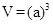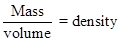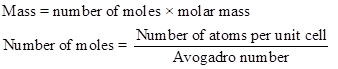Chapter 12, Problem 11PS

Chapter
Section
Textbook Problem

Potassium iodide has a face-centered cubic unit cell of iodide ions with potassium ions in octahedral holes. The density of KI is 3.12 g/cm3. What is the length of one side of the unit cell? (Ion sizes are found in Figure 7.11.)

Interpretation Introduction

Interpretation:

The length of one side of unit cell has to be calculated.

Concept introduction:

To find the volume of the atom is given below,The density of the unit cell is calculated as follows,Explanation

Therefore,

Still sussing out bartleby?

Check out a sample textbook solution.

See a sample solution

The Solution to Your Study Problems

Bartleby provides explanations to thousands of textbook problems written by our experts, many with advanced degrees!

Get Started

Find more solutions based on key concepts